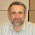## Friday, August 3, 2012

### Horizon Plots in Base Graphics

for background please see prior posts More on Horizon Charts, Application of Horizon Plots, Horizon Plot Already Available, and Cubism Horizon Charts in R

There are three primary graphics routes in R (base graphics, lattice, and ggplot2), and each have their zealots.  Last time in More on Horizon Charts, I used lattice and latticeExtra. This time we will build horizon plots in base graphics, and I was pleased with the result. Unfortunately, there is one small issue in that the points of change from positive to negative overlap.  Please let me know if you have a solution. Thanks to helpful readers for their comments and code changes, this is now fixed.

Now, let’s implement a for loop and mirror the negative values.

With horizon chart functionality in base graphics, hopefully we can add this type now to other packages.  Here is a potential example using quantmod.

R code in GIST (do raw for copy/paste)

1.You key issue in how you defined the polygons. In the data you have, for example:

2002-05-30 as 0.010353965
and
2002-06-29 as -0.013995566

However in creating the positive band you forced the second value to be zero instead of -0.013... and in creating the negative band you forced the first value to be zero instead of 0.0103...

So your polygons do not match the data. To get the graph working right you will have to determine the crossover point - which is not the value of x of either of the two dates but for some point between those two values. Then use that point to define the polygons.

The effect of the difference is easy to see if you insert the code

#draw Actual polygon
polygon(
index(x)[c(1,1:n,n)],
c(0,coredata(x),0),
col="red")

after you make the first plot with the positive and negative polygons.

2.simpler and no overlap:

horplot=function(x, nBrakes= 6, border = NA) {
nBrakes= 2 * floor(nBrakes/2) # nBrakes should be even

h= hist(x, br= nBrakes, plot= T)
dx= diff(h\$breaks)

n= NROW(x)

plot(index(x), coredata(x), ylim=c(0,dx), type="n")
par(usr=c(index(x),index(x)[n],0,dx)) # 0-margines

for(i in 1:(nBrakes/2)){
polygon(
c(index(x), index(x), index(x)[n]),
c(0, x - h\$breaks[i+ nBrakes/2-1], 0),
col= i + nBrakes/2, border = border
)
polygon(
c(index(x), index(x), index(x)[n]),
c(0,-x + h\$breaks[i], 0),
col= i , border = border
)
}
}

horplot(x, 6)
horplot(x, 6, border =T)